AETv2: AutoEncoding Transformations for Self-Supervised Representation Learning by Minimizing Geodesic Distances in Lie Groups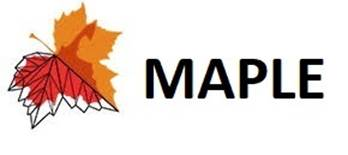Guo-Jun Qi

Laboratory for MAchine Perception and LEarning (MAPLE)

Abstract:  Self-supervised learning by predicting transformations has demonstrated outstanding performances in both unsupervised and (semi-)supervised tasks. Among the state-of-the-art methods is the AutoEncoding Transformations (AET) by decoding transformations from the learned representations of original and transformed images. Both deterministic and probabilistic AETs rely on the Euclidean distance to measure the deviation of estimated transformations from their groundtruth counterparts. However, this assumption is questionable as the group of underlying transformations often reside on a curved manifold rather staying in the flat Euclidean space. For this reason, we should use the geodesic to characterize how an image transform along the manifold of a Lie group, and adopt its length to measure the loss between two transformations. Particularly, we present to autoencode the homography Lie group PG(2) that contains a rich family of spatial transformations to learn image representations. Moreover, we make an estimate of the intractable Riemannian logarithm by projecting PG(2) to a subgroup of rotation transformations SO (3)\$ that allows the matrix logarithm. Experiments demonstrate the proposed AETv2 model greatly outperforms its previous version as well as the other state-of-the-art self-supervised models in multiple representation learning tasks.

## Formulation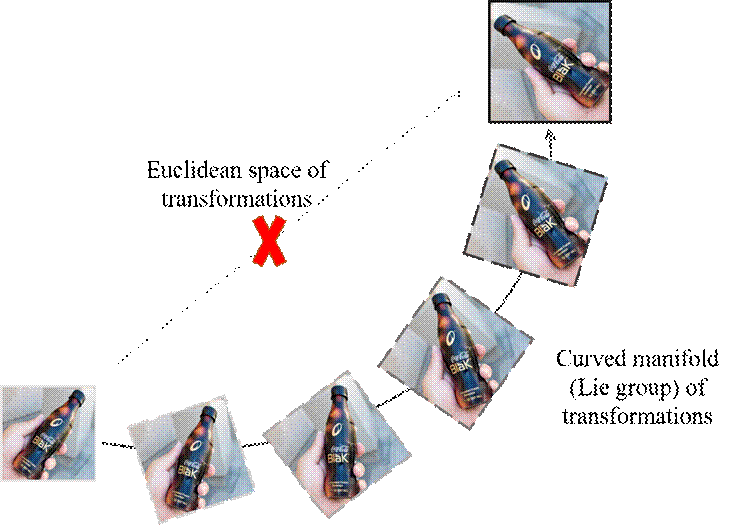Figure 1: The deviation between two transformations should be measured along the curved manifold (Lie group) of transformations rather than through the forbidden Euclidean space of transformations.

Unlike the first AET , the AETv2 is a self-trained model by minimizing the geodesic distance between two transformations, since a transformation continuously evolves to another transformation along the manifold of transformations rather than through the forbidden Euclidean space of transformations as shown in Figure 1.

For this purpose, we propose to train the AETv2 in the Lie group of transformations, by minimizing the following geodesic distance between the grountruth through the Riemannian logarithm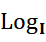at the identity transformation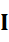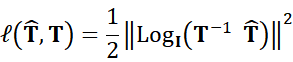However, directly computing the Riemannian logarithm can be intractable for many types of transformations.  For example, the group of homography transformations does not have a closed-form Riemannian logarithm, and we instead choose to project them into a subgroup SO(3) of 3D rotations in homogeneous coordinate space for images, as shown in Figure 2 below. This eventually results in the following approximate loss defined over the projected SO(3) subgroup,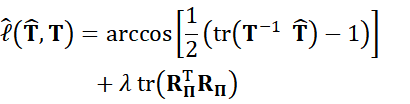where we combine the geodesic distance of the first term with the projection distance in the second term, and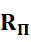is the projection residual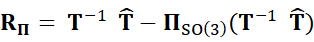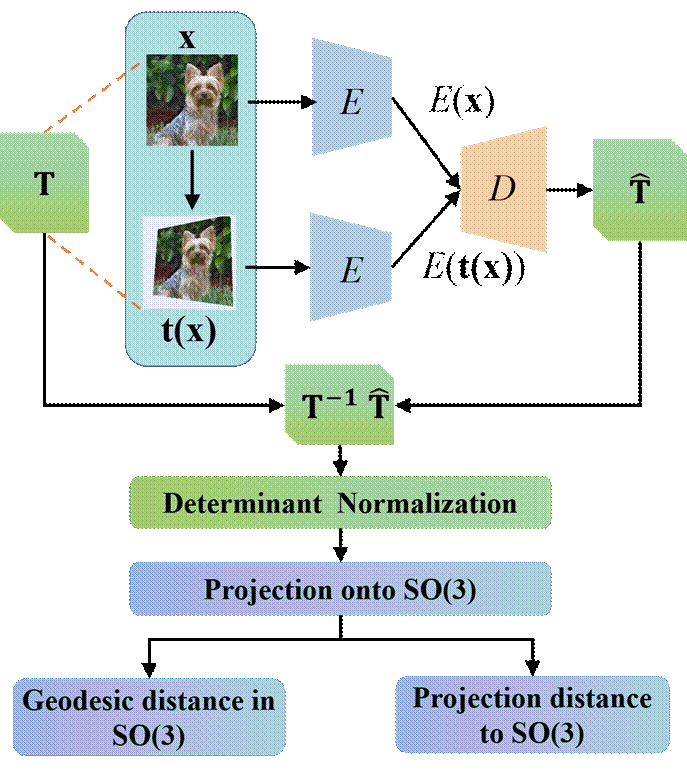Figure 2: Illustration of how the AETv2 is self-trained end-to- end. The output matrix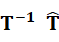from the transformation decoder is normalized to have a unit determinant, and the projection ofonto SO(3) follows to compute the geodesic distance and the projection distance train the model.

## Results

Table 1 below reports the experiment results on CIFAR-10 with a three-layer nonlinear classifier being trained on top of the first two blocks that are pretrained by AETv2.

We also train Alexnet by AET and evaluate the performance of learned representations based on Conv4 and Conv 5 output feature maps.  The proposed AETv2 can significantly close the performance gap with the fully supervised network as shown in Table 3 in the paper below.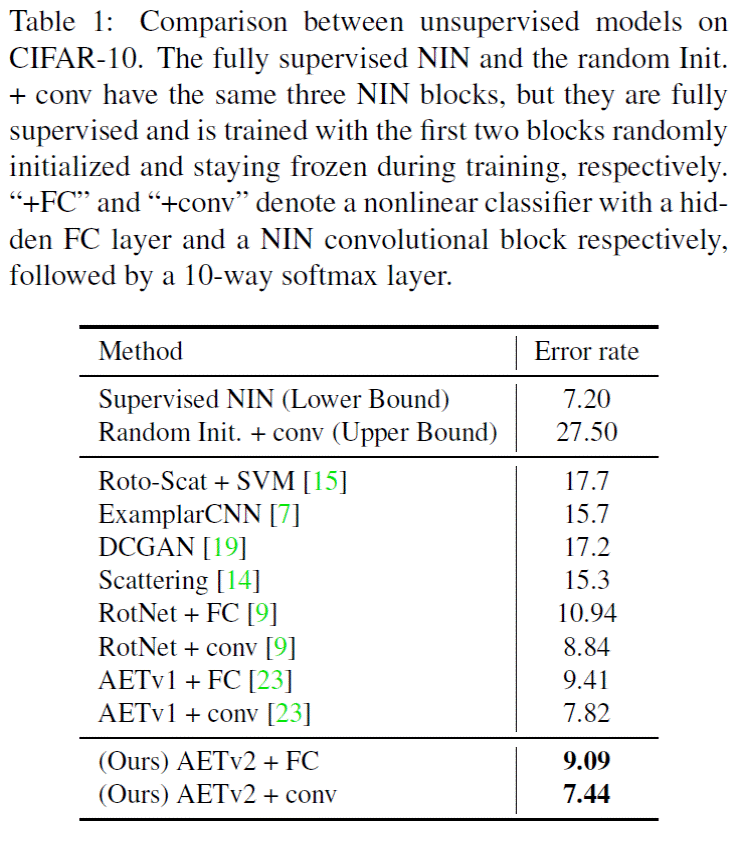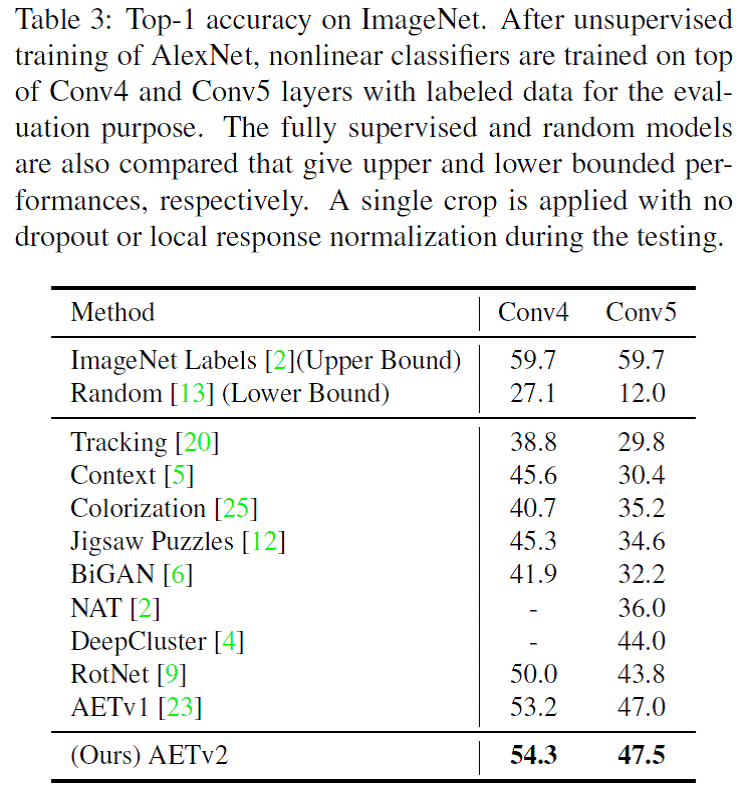More experiment results on ImageNet and Places datasets can be found in our paper . The source code will be released later.

## Citation

 Liheng Zhang, Guo-Jun Qi, Liqiang Wang, Jiebo Luo. AET vs. AED: Unsupervised Representation Learning by Auto-Encoding Transformations rather than Data, in Proceedings of IEEE Conference on Computer Vision and Pattern Recognition (CVPR 2019), Long Beach, CA, June 16th - June 20th, 2019.  [ pdf ]

 Feng Lin, Haohang Xu, Houqiang Li, Haohang Xu, and Guo-Jun Qi, AETv2: AutoEncoding Transformations for Self-Supervised Representation Learning by Minimizing Geodesic Distances in Lie Groups.

November 16, 2019